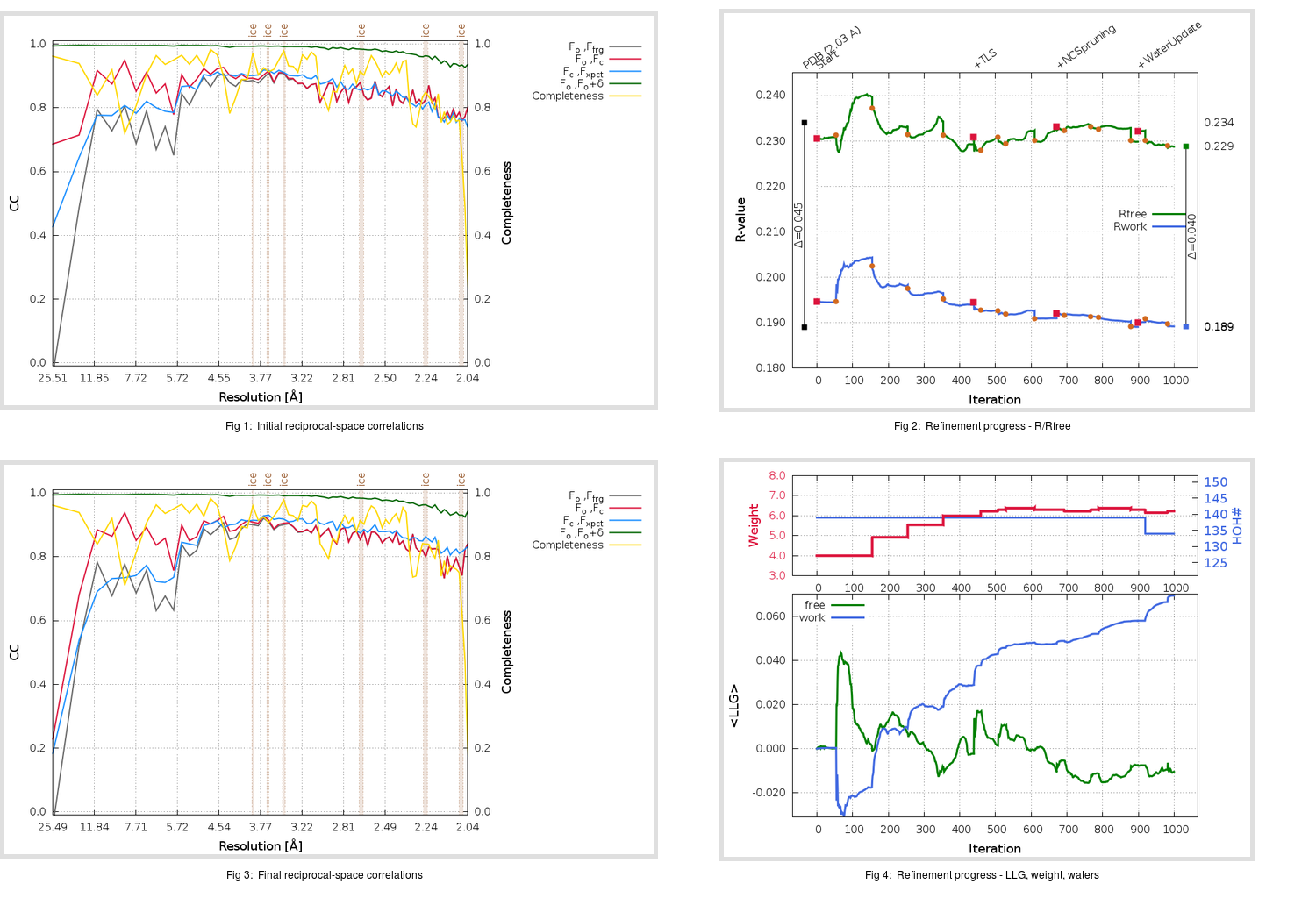Content:

```    Diffraction limits & principal axes of ellipsoid fitted to diffraction cut-off surface:
1.953         0.9688   0.2304  -0.0910       0.950 a* + 0.279 b* + 0.139 c*
1.984        -0.2428   0.9561  -0.1642      -0.196 a* + 0.965 b* - 0.176 c*
2.093         0.0492   0.1812   0.9822       0.023 a* + 0.105 b* + 0.994 c*
```

## Deposited

` `
 Date deposited Date data collection Resolution R, Rfree 20200224 20200221 2.03 0.1860 0.2340

Molprobity (CCP4 7.0 version) summary:

```Ramachandran outliers =   0.00 %
favored =  98.48 %
Rotamer outliers      =   2.52 %
C-beta deviations     =     0
Clashscore            =   1.16
RMS(bonds)            =   0.0041
RMS(angles)           =   0.97
MolProbity score      =   1.13
Resolution            =   2.03
R-work                =   0.1860
R-free                =   0.2340
```

```Number of waters      =   139

<B> (all atoms) =   24.57 ( sd =    9.77 ) for       2715 non-hydrogen atoms
<B>   (protein) =   23.84 ( sd =    9.10 ) for       2526 non-hydrogen atoms
<B>     (water) =   29.20 ( sd =    6.65 ) for        139 non-hydrogen atoms
<B>    (others) =   48.80 ( sd =   14.17 ) for         50 non-hydrogen atoms

B min/max       (all non-hydrogen atoms) =   10.40 /   73.17
B min/max   (protein non-hydrogen atoms) =   10.40 /   66.02
B min/max     (water non-hydrogen atoms) =   17.54 /   46.87
B min/max     (other non-hydrogen atoms) =   34.07 /   73.17
```

## BUSTER (re-)refinement

` `

Molprobity (CCP4 7.0 version) summary:

```Ramachandran outliers =   0.00 %
favored =  98.48 %
Rotamer outliers      =   1.44 %
C-beta deviations     =     0
Clashscore            =   2.90
RMS(bonds)            =   0.0113
RMS(angles)           =   1.49
MolProbity score      =   1.20
Resolution            =   2.03
R-work                =   0.1892
R-free                =   0.2288
```

```Number of waters      =   134

<B> (all atoms) =   22.47 ( sd =    8.21 ) for       2710 non-hydrogen atoms
<B>   (protein) =   21.70 ( sd =    7.54 ) for       2526 non-hydrogen atoms
<B>     (water) =   30.65 ( sd =    9.18 ) for        134 non-hydrogen atoms
<B>    (others) =   39.45 ( sd =    7.82 ) for         50 non-hydrogen atoms

B min/max       (all non-hydrogen atoms) =    9.42 /   74.06
B min/max   (protein non-hydrogen atoms) =    9.42 /   58.70
B min/max     (water non-hydrogen atoms) =   14.99 /   74.06
B min/max     (other non-hydrogen atoms) =   31.30 /   54.67
```

Refinement progression:Results:

` `
 File Remark 6VXS_aB_refine.01_04_refine.pdb.gz exact refinement commands are in header 6VXS_aB_refine.01_04_refine.mtz.gz including original deposited data and several re-refinement map coefficients 6VXS_aB_refine.01_04_BUSTER_model.cif.gz including any non-standard compound restraints 6VXS_aB_refine.01_04_BUSTER_refln.cif.gz